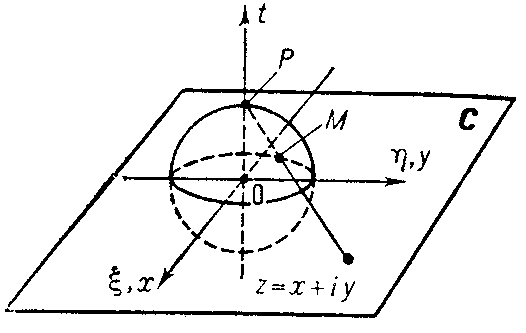# Riemann sphere

A sphere in the Euclidean space $\mathbf R ^ {3} ( \xi , \eta , t)$ onto which the extended complex plane $\overline{\mathbf C}\;$ is conformally and one-to-one transformed under stereographic projection. For example, the unit sphere

$$S _ {2} = \{ {( \xi , \eta , t) \in \mathbf R ^ {3} } : { \xi ^ {2} + \eta ^ {2} + t ^ {2} = 1 } \}$$

can be taken as the Riemann sphere and the plane $\overline{\mathbf C}\;$ can be identified with the plane $t = 0$ such that the real axis coincides with the axis $\eta = 0, t = 0$ and the imaginary axis with the axis $\xi = 0, t = 0$ (see Fig.).Figure: r082010a

Under stereographic projection to each point $z = x + iy \neq \infty$ there corresponds the point $M( \xi , \eta , t) \neq P( 0, 0, 1)$ obtained as the point of intersection of the ray drawn from the north pole of the sphere, $P( 0, 0, 1)$, to the point $z$ with the sphere $S _ {2}$; the north pole $P( 0, 0, 1)$ corresponds to the point at infinity, $z = \infty$. Analytically this relation can be expressed by the formulas

$$\tag{* } \xi + i \eta = \ \frac{2z}{| z | ^ {2} + 1 } ,\ \ t = \frac{| z | ^ {2} - 1 }{| z | ^ {2} + 1 } ,\ \ z = \frac{\xi + i \eta }{1-t }.$$

In other words, the correspondence (*) determines a differentiable imbedding of the one-dimensional complex projective space $\mathbf C P ^ {1}$ into the space $\mathbf R ^ {3}$ in the form of the sphere $S _ {2}$. In many questions of the theory of functions, the extended complex plane is identified with the Riemann sphere. The exclusive role of the point at infinity of the plane $\overline{\mathbf C}\;$ may be dispensed with if the distance between two points $z, w \in \overline{\mathbf C}\;$ is taken to be the chordal, or spherical, distance $\chi ( z, w)$ between their images $M, N \in S _ {2}$:

$$\chi ( z, w) = \frac{2| z- w | }{\sqrt {| z | ^ {2} + 1 } \sqrt {| w | ^ {2} + 1 } } ,$$

$$\chi ( z, \infty ) = \frac{2}{\sqrt {| z | ^ {2} + 1 } } .$$

A higher-dimensional complex projective space $\mathbf C P ^ {n}$, $n > 1$, can be imbedded into the space $\mathbf R ^ {n(n+ 2)}$ by a complex $n$-dimensional stereographic projection, generalizing the formulas (*) (see ).

How to Cite This Entry:
Riemann sphere. Encyclopedia of Mathematics. URL: http://encyclopediaofmath.org/index.php?title=Riemann_sphere&oldid=52811
This article was adapted from an original article by E.D. Solomentsev (originator), which appeared in Encyclopedia of Mathematics - ISBN 1402006098. See original article